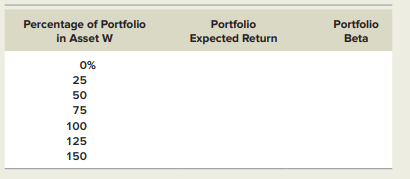### Create an Account

Home / Questions / Using the SML Asset W has an expected return of 114 percent and a beta of 1 18 If the ris...

# Using the SML Asset W has an expected return of 114 percent and a beta of 1 18 If the risk free rate is 3 15 percent complete the following table for portfolios of Asset W and a risk free asset

Using the SML. Asset W has an expected return of 11.4 percent and a beta of 1.18. If the risk-free rate is 3.15 percent, complete the following table for portfolios of Asset W and a risk-free asset. Illustrate the relationship between portfolio expected return and portfolio beta by plotting the expected returns against the betas. What is the slope of the line that results?May 25 2020 View more View LessSubscribe To Get Solution